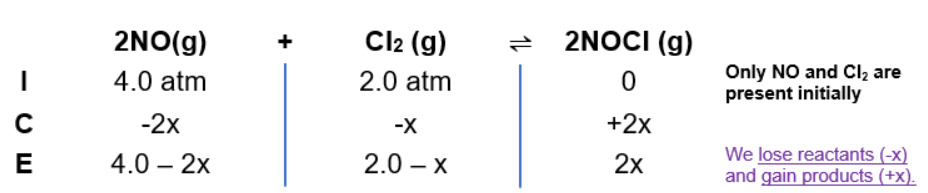# Problem: Calculate the pressures of NO, Cl 2, and NOCl in an equilibrium mixture produced by the reaction of a starting mixture with 4.0 atm NO and 2.0 atm Cl2. (Hint: KP is small; assume the reverse reaction goes to completion then comes back to equilibrium.)2NO(g) + Cl2(g) ⇌ 2NOCl(g)             K P = 2.5 × 10-3

###### FREE Expert Solution

2 NO(g) + Cl2(g)  2 NOCl(g)

When dealing with equilibrium and Kp:

Kp → equilibrium units in terms of pressure
Kp is an equilibrium expression:

$\overline{){{\mathbf{K}}}_{{\mathbf{p}}}{\mathbf{=}}\frac{\mathbf{products}}{\mathbf{reactants}}{\mathbf{=}}\frac{{{\mathbf{P}}_{\mathbf{NOCl}}}^{\mathbf{2}}}{{{\mathbf{P}}_{\mathbf{NO}}}^{\mathbf{2}}\mathbf{×}{\mathbf{P}}_{{\mathbf{Cl}}_{\mathbf{2}}}}}$

ICE Chart:95% (100 ratings)###### Problem Details

Calculate the pressures of NO, Cl 2, and NOCl in an equilibrium mixture produced by the reaction of a starting mixture with 4.0 atm NO and 2.0 atm Cl2. (Hint: KP is small; assume the reverse reaction goes to completion then comes back to equilibrium.)
2NO(g) + Cl2(g) ⇌ 2NOCl(g)             K P = 2.5 × 10-3

Frequently Asked Questions

What scientific concept do you need to know in order to solve this problem?

Our tutors have indicated that to solve this problem you will need to apply the ICE Chart concept. You can view video lessons to learn ICE Chart. Or if you need more ICE Chart practice, you can also practice ICE Chart practice problems.

What professor is this problem relevant for?

Based on our data, we think this problem is relevant for Professor Sharpe Elles' class at KU.

What textbook is this problem found in?

Our data indicates that this problem or a close variation was asked in Chemistry - OpenStax 2015th Edition. You can also practice Chemistry - OpenStax 2015th Edition practice problems.